# Dynamic Asset Allocation For Practitioners

|
Includes: DBC, EEM, EWJ, GLD, ICF, IEF, IEV, RWX, TLT, VTI
by: GestaltU

A (Very) Short History of Dynamic Asset Allocation

The field of tactical or dynamic asset allocation has grown dramatically since Mebane Faber published what is perhaps the first broadly accessible paper on the topic in 2007, 'A Quantitative Approach to Tactical Asset Allocation'. Faber's original paper utilized a simple 10 month moving average as a signal to move into or out of a basket of 5 major global asset classes. Over the period 1970 through the paper's 2009 update, this technique generated better returns than any of the individual assets in the sample universe - U.S. and EAFE stocks, U.S. real estate, Treasuries and commodities - and with substantially lower risk than the equal weight basket or a 60/40 stock/Treasury portfolio.

In 2009 Faber published a follow-up paper called 'Relative Strength Strategies for Investing' which introduced the concept of price momentum as a way to distinguish between strong and weak assets in the portfolio. That paper applied an intuitive method of capturing asset class momentum that involved averaging each asset's rate of change (ROC) across five lookback horizons, specifically 1, 3, 6, 9 and 12 months. By averaging across lookback horizons, this approach captures momentum at multiple periodicities, and also identifies acceleration by implicitly weighting near-term price moves more heavily than price moves at longer horizons.

In May of 2012 we published a whitepaper entitled, "Adaptive Asset Allocation: A Primer", a quantitative systematic methodology integrating the simple ROC based momentum concepts introduced in Faber's 'Relative Strength' paper with techniques derived from the portfolio optimization literature. Specifically, the paper explained how applying a minimum variance optimization overlay to a portfolio of high momentum assets serves to stabilize and strengthen both absolute and risk-adjusted portfolio performance.

Article Series

We are going to range far and wide in our exploration of global dynamic asset allocation. This article, the first in our series, will explore a variety of methods to rank assets based on price momentum. The second article will introduce several approaches to rank assets based on risk-adjusted momentum measures. The third article will introduce a framework for thinking about portfolio optimization, including several heuristic and formal optimization methods.

Our fourth article will discuss ways of combining the best facets of momentum with the best techniques for portfolio optimization to offer a coherent framework for global dynamic asset allocation. The objective here will be robustness and logical coherence rather than utilizing optimization for best in-sample simulation performance.

Lastly, we are considering introducing some ensemble concepts and adaptive frameworks as a cherry on top, but we aren't sure how far we want to go yet, so we'll just get started and see where it takes us.

The following illustrates the proposed framework for this article series:

Methodology

This first article will explore a variety of methods for identifying trend strength for asset allocation, with the goal of comparing and contrasting the various methods under different assumptions of portfolio concentration and asset universe specifications.

We take the position that portfolio concentration is a source of potential data mining bias because the results for dynamic asset allocation approaches can vary widely depending on the number of top assets that are held in the portfolio at each rebalance. Some approaches do better with more concentration, and others with less. We will test with concentrations of top 2, 3, 4 and 5 assets and average the results.

The asset universe can serve as a source of potential 'curve fitting' as well, as it is easy and compelling to want to remove assets from the universe that drag down returns in simulation, or add assets with strong results over the backtest horizon.

To avoid this trap, we run our simulations on a diversified universe of 10 global asset classes, as well as ten other asset universes where we drop one of the ten original assets. This helps to control for the chance that strong performance is simply the result of one dominant asset class over the period.

The ten asset classes we will use for all testing are:

• Commodities (DB Liquid Commodities Index: DBC)
• Gold (NYSEARCA:GLD)
• U.S. Stocks (Fama French top 30% by market capitalization: VTI)
• European Stocks (Stoxx 350 Index: IEV)
• Japanese Stocks (MSCI Japan: EWJ)
• Emerging Market Stocks (MSCI EM: EEM)
• U.S. REITs (Dow Jones U.S. Real Estate Index: ICF)
• International REITs (Dow Jones Int'l Real Estate Index: RWX)
• Intermediate Treasuries (Barclays 7-10 Year Treasury Index: IEF)
• Long Treasuries (Barclays 20+ Year Treasury Index: TLT)

Importantly, this article is not about parameter optimization; for all tests we use the exact same lookback parameter lengths (where applicable) to avoid the distraction of searching for a priori local parameter optima which will almost certainly not prove to be true optima out of sample.

It is important to decide how we will evaluate the relative efficacy of the various approaches before we start testing. For each strategy we will show the average statistics for all simulations with 2, 3, 4, and 5 holdings, and across all 11 asset universes. Recall that we are testing the full 10 asset class universe, as well as 10 other 9 asset class universes where one of the original assets is removed. So the statistics for each strategy will actually represent an average (median) of 44 simulations (4 portfolio concentrations x 11 universes). We will then present modified histograms to illustrate the range of outcomes for each strategy. This represents a rare test of robustness across methodologies.

Toward the bottom of this article, we demonstrate how combining all of the indicators into a naive ensemble delivers better performance than any of them individually.

Momentum Metrics

For the purpose of this article we used 8 indicators for measuring trend strength. All of the metrics rank assets at monthly rebalance periods based on an average of values observed over the lookback windows described above, which were chosen to be consistent with Mebane Faber's original momentum paper.

The intuition behind testing a variety of momentum techniques relates to the ability of different measures to stabilize the estimate using simple or advanced ensemble process.

The following list describes the mechanics of each method of momentum calculation, where t is the current date, n is the lookback parameter, and N is the number of assets in the testing universe. Note that each indicator is calculated at each of the 5 lookbacks, and then the indicators are averaged across lookbacks to generate the final measure.

• Total return - this is the most common measure of momentum, where assets are ranked on their historical total returns.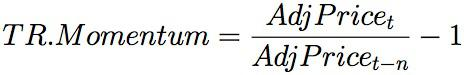• SMA Differential - this technique uses the differential between a shorter term and longer term moving average as the momentum measure. We used our standard parameters to define the length of the longer SMAs. The length of corresponding short SMAs was simply 1/10th of the length of the longer SMA. So for example, for the 120 day parameter, we measured the differential between the 120 day SMA and the 12 day SMA.
• Price to SMA Differential - similar to SMA Differential, except that this technique uses the differential between the current price and the n-day SMA rather than using a shorter moving average.
• SMA Instantaneous Slope - For this metric we derived the instantaneous slope of each moving average. Essentially this measures the rate of change of each SMA using the difference between yesterday's SMA and today's SMA.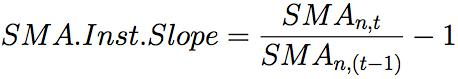• Price Percent Rank - This metric captures the location of the current price relative to the security's range over each lookback period. The lowest price over the period would have a rank of 1, while the highest would have a rank of 100. The median price over the period would have a rank of 50.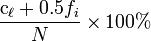• Z-Score - Analogous to the Price Percent Rank, z-score captures the magnitude that the current price deviates from the average price over the period.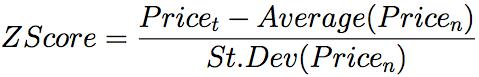• Z-Distribution - This method transforms the z-score to a percentile value on the cumulative normal distribution. Under this framework the trend strength measure will accelerate in magnitude as the price strays further away from the mean. The function to perform this translation is complicated, but it can be easily generated in Excel using the Norm.S.Dist(z, TRUE) function.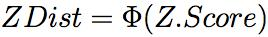• T-Distribution - The normal distribution is valid when the sample size is large enough so that the sample is likely to be representative of the population. Under conditions where the sample size is small and the parameters that describe the distribution are unknown, a more appropriate choice is the Student's t-distribution. The t-distribution transforms a t-score into a percentile given the number of degrees of freedom. The degrees of freedom are equal to (n - 1).

It is worth noting that the SMA-differential approaches described above are related to typical moving average crossover systems applied in trend following. The critical difference in our proposed framework is that, unlike trend following approaches, which measure the 'state' of a trend, our momentum indicators measure the 'strength' of a trend. Crossover systems are either long or short (triple crossovers can be neutral too), which means they are 'binary' variables, whereas the momentum indicators provide discrete variables that allow us to compare the relative strength of the trends.

Importantly, because we measure trend strength across five lookback time horizons, the cross sectional measure of price momentum needs to be standardized. It is silly to average an annualized 20 day ROC with a 250 day ROC because the 20 day ROC will deliver much more extreme values, on average, than the 250 day ROC, and will dominate the momentum measure commensurately.

There are a number of ways to standardize the momentum measure across lookbacks, but the method we used was to calculate each asset's proportion of total absolute cross sectional momentum across all assets over each lookback horizon, holding the sign constant.

Again, standardized momentum scores are then averaged across all lookbacks to determine the final momentum score for each asset.

Results

Table 1. displays the salient statistics for tests of each of the momentum methods (indicators) described above. Each cell describes the median performance across all 44 combinations (holding 2 - 5 positions, 11 universe combinations) that we tested for each methodology.

Chart 1. Median performance summary

Data sources: Bloomberg

The instantaneous slope method seems to deliver the best median performance statistics all around, with the highest overall returns, the highest Sharpe, and the lowest median Maximum Drawdown of all methods. But the median is just one point on the distribution; let's see what the range of outcomes looks like for each system.

Performance Distribution

Charts 1 through 9 below show all 44 of the equity lines (4 concentrations x 11 universe combinations) that were used to calculate the median performance measures in Table 1 for each momentum indicator. The first highlighted chart shows all 352 equity lines derived from all 44 portfolio combinations across all 8 indicators.

Charts 1 - 9: Equity lines for indicators across 44 universe/concentration combinations

Source: Bloomberg

A few observations stand out from these charts. First, they all look a little different, with waves of surges and drawdowns occurring at slightly different times across momentum measures, though all of them show a drawdown in 2008. Second, notice that in some charts the equity lines all cluster together in a narrow range - z-score and instantaneous slope stand out in this respect - while others exhibit quite a wide range of outcomes - price to SMA differential and SMA differential for example.

Charts 10 through 43 below quantify the distribution of return, Sharpe, and Maximum Drawdown outcomes across all 44 versions of each momentum system using a cumulative histogram. In our opinion, the most realistic way to evaluate the performance of a system is on the basis of performance statistics near the bottom of the distribution. The worst outcome could be an outlier, but traditional tests of statistical significance focus on 5th percentile outcomes, so that is where we focus our attention. In each series of charts, we have highlighted the approach with the best results at the 5th percentile.

Charts 10 - 43: Distribution of performance metrics across 44 universe/concentration combinations.

Range of CAGR by Indicator

Source: Bloomberg

Range of Sharpe(0%) by Indicator

Source: Bloomberg

Range of Max Drawdowns by Indicator

Source: Bloomberg

Note that in terms of returns, Sharpe ratio, and Maximum Drawdown, the instantaneous slope demonstrates the best performance at the 5th percentile, confirming what we observed in the median summary in Table 1. (You will note that the numbers in the 50% column of the charts above are the same as the numbers in Table 1 summary, as the median is simply the 50th percentile value).

Charts 24 through 26 show the average performance of each methodology with portfolio concentrations of 2 holdings through 5 holdings across all 11 asset universes tested. It is interesting to see that, while more concentrated portfolios tend to deliver higher returns, the highest Sharpe ratios are derived from portfolios with 3 or 4 holdings, and these more diversified portfolios tend toward much lower drawdowns as well.

Chart 24. Average indicator returns across 11 asset universe combinations with different portfolio concentration

Source: Bloomberg

Chart 25. Average indicator return/risk ratios across 11 asset universe combinations with different portfolio concentration

Source: Bloomberg

Chart 26. Average indicator Max Drawdowns across 11 asset universe combinations with different portfolio concentration

Source: Bloomberg

Indicator Diversification

We know from charts 1 - 9 that the different momentum indicators, universe and concentration combinations all deliver slightly different results, where equity rises and falls at slightly different rates. But how different is the performance across indicators really?

Matrix 1. shows the correlations between daily returns for all indicator combinations. The daily returns for all universe and concentration combinations were averaged to generate the final return series for each indicator.

Matrix 1. Pairwise correlations between indicators (average of 44 combinations for each indicator)

Source: Bloomberg

The correlations range from about 0.8 between the t-distribution method and the ROC and instantaneous slope methods, to 0.99 between instantaneous slope and ROC. The average pairwise correlation is 0.936. With correlations so high, to what extent can we take advantage of the different methods to create a diversified system composed of all 352 combinations? Chart 27 and Table 2. give us the answer.

Chart 27. Aggregate Index of all 352 indicator/universe/concentration combinations, equal weight.

Source: Bloomberg

Table 2. Summary statistics for Aggregate Index

Source: Bloomberg

Despite the high average correlations between the different momentum systems, the aggregate equity line provides a material boost to all risk adjusted statistics. While the returns are about 1% below the returns derived from the best individual indicators, the Sharpe(0%) ratio is better than all of them. Further, the average volatility of the 8 individual systems is 12.42%, while the volatility of the aggregate system is the lowest of all at just 11.1%. Lastly, the aggregate system exhibits the lowest drawdowns and the highest percentage of positive rolling 12-month periods.

Obviously it is impractical to run 352 models in parallel, even if they are all closely related. Moreover, this approach is far from the best method to aggregate all of the information from the different indicators; we will touch on different methods of aggregation in our fourth installment of this series. However, there is clearly value in finding ways to blend various momentum factors to create a more stable allocation model.

Conclusions and Next Steps

In this article we have explored a variety of methods to measure the price momentum of a universe of asset classes for the purpose of creating global dynamic asset allocation models. Given our objective to avoid as much optimization as possible, we tested each momentum method using 4 different levels of portfolio concentration, and 11 slightly modified asset class universes. This approach provided 44 distinct tests for each indicator, which allowed us to investigate the stability of each indicator across parameters.

Among the individual momentum indicators we tested, the instantaneous slope method delivered the best performance in terms of median returns, Sharpe(0%), drawdowns and percent positive 12-month periods. This method also delivered the most stable results at the 5th percentile (95th percentile for drawdowns).

We investigated the impact of different levels of portfolio concentration on performance and discovered, perhaps not surprisingly, that more concentrated portfolios deliver stronger returns, while some diversification does improve risk-adjusted outcomes.

We examined the correlations between the indicator systems and determined that they are all closely related, with average pairwise correlations of about 0.93. However, even with such high average correlations, aggregating the systems into one system composed of all 352 indicator/concentration/ universe combinations delivered the most stable results of all.

Article 2 in our series will perform a similar analysis of several risk-adjusted momentum measures, such as Sharpe ratio, Omega ratio, and Sortino Ratio. As in this article, the Article 2 will hold all portfolio positions in equal weight, but Article 3 will introduce methods to optimize the weights of portfolio holdings to further improve absolute and risk adjusted returns - quite significantly.

We are just scratching the surface of what is possible with tactical alpha. Chart 27 and Table 3. offer a glimpse of what's to come. Stay tuned.

Chart 27. Mystery system

Source: Bloomberg

Table 3. Mystery system stats

Disclosure: I have no positions in any stocks mentioned, and no plans to initiate any positions within the next 72 hours. I wrote this article myself, and it expresses my own opinions. I am not receiving compensation for it. I have no business relationship with any company whose stock is mentioned in this article.